# CE 3372 Water Systems Design Closed Conduit HydraulicsI

• Slides: 33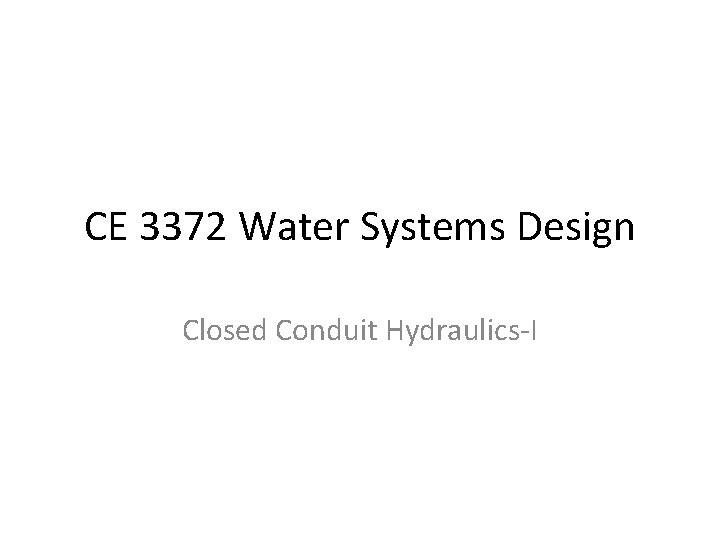CE 3372 Water Systems Design Closed Conduit Hydraulics-I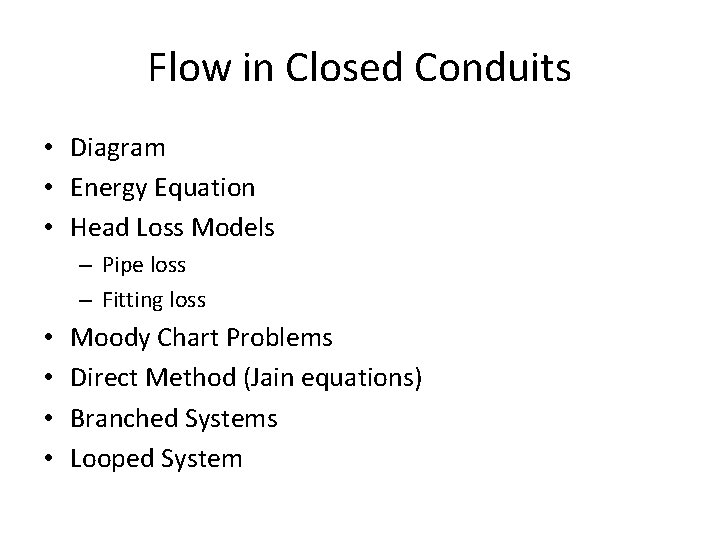Flow in Closed Conduits • Diagram • Energy Equation • Head Loss Models – Pipe loss – Fitting loss • • Moody Chart Problems Direct Method (Jain equations) Branched Systems Looped SystemDiagramDiagram Lift Station Suction Side Discharge SideMean Section Velocity • In most engineering contexts, the mean section velocity is the ratio of the volumetric discharge and cross sectional area. • The velocity distribution in a section is important in determining frictional losses in a conduit.Energy Equation • The energy equation relates the total dynamic head at two points in a system, accounting for frictional losses and any added head from a pump.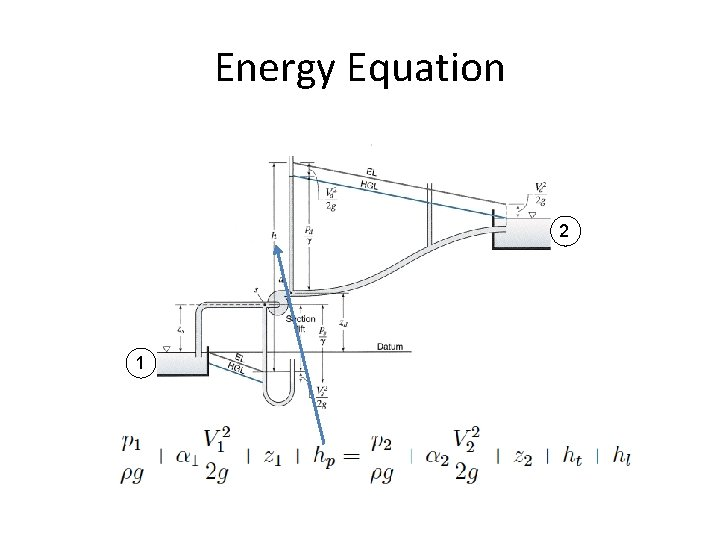Energy Equation 2 1Head Loss Models • Darcy-Weisbach • Hazen-Williams • Chezy-ManningsDarcy-Weisbach • Frictional loss proportional to – Length, Velocity^2 • Inversely proportional to – Cross sectional area • Loss coefficient depends on – Reynolds number (fluid and flow properties) – Roughness height (pipe material properties)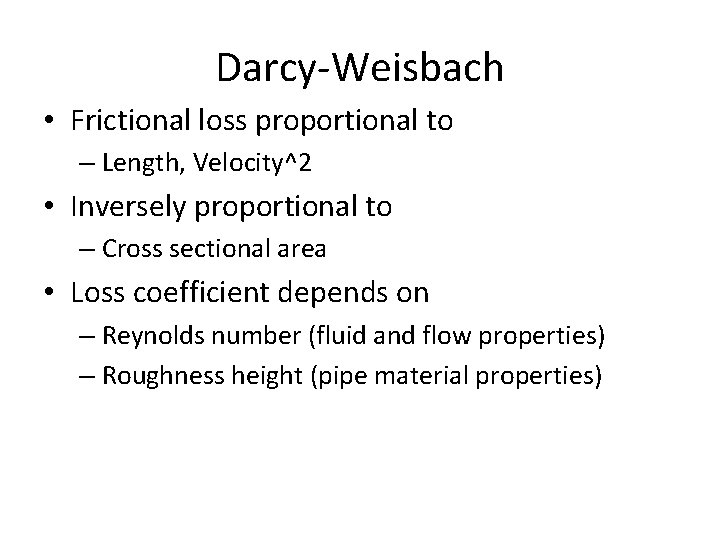Darcy-Weisbach • Frictional loss proportional to – Length, Velocity^2 • Inversely proportional to – Cross sectional area • Loss coefficient depends on – Reynolds number (fluid and flow properties) – Roughness height (pipe material properties)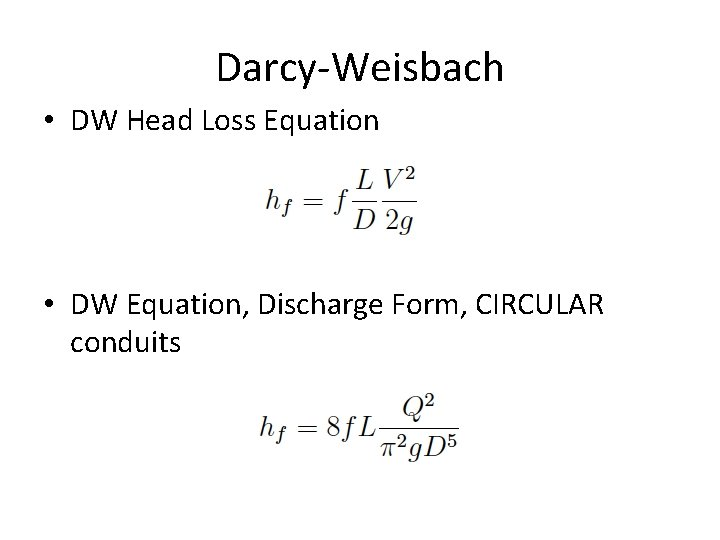Darcy-Weisbach • DW Head Loss Equation • DW Equation, Discharge Form, CIRCULAR conduitsHazen-Williams • Frictional loss proportional to – Length, Velocity^(1. 8) • Inversely proportional to – Cross section area (as hydraulic radius) • Loss coefficient depends on – Pipe material and finish • WATER ONLY!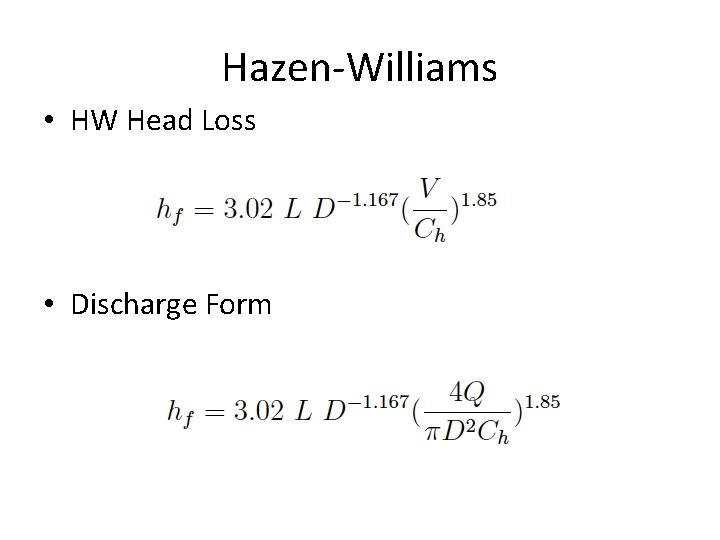Hazen-Williams • HW Head Loss • Discharge FormHydraulic Radius • HW is often presented as a velocity equation using the hydraulic radius • The hydraulic radius is the ratio of cross section flow area to wetted perimeter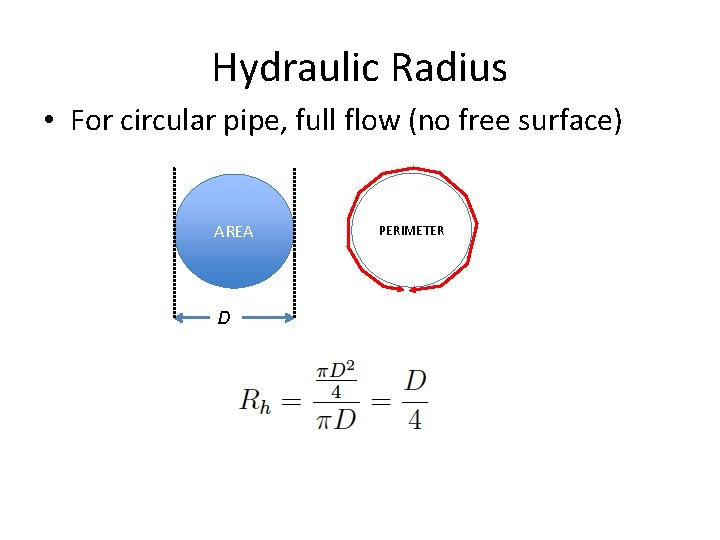Hydraulic Radius • For circular pipe, full flow (no free surface) AREA D PERIMETERChezy-Manning • Frictional loss proportional to – Length, Velocity^2 • Inversely proportional to – Cross section area (as hydraulic radius) • Loss coefficient depends on – Material, finish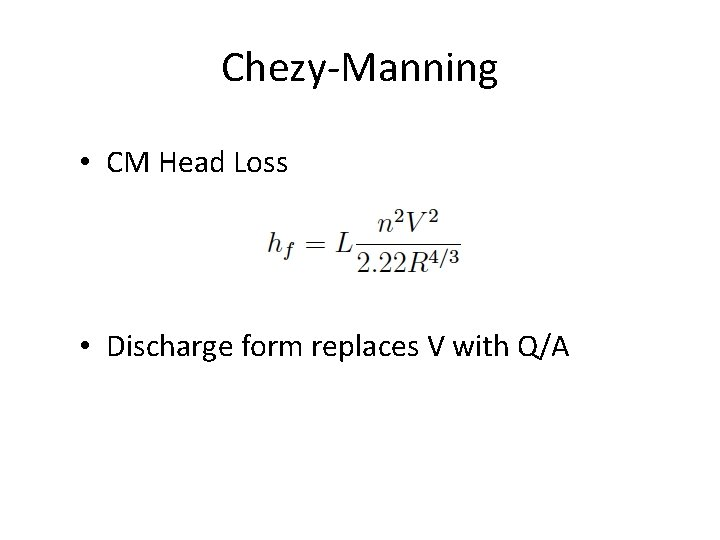Chezy-Manning • CM Head Loss • Discharge form replaces V with Q/AFitting (Minor) Losses • Fittings, joints, elbows, inlets, outlets cause additional head loss. • Called “minor” loss not because of magnitude, but because they occur over short distances. • Typical loss model is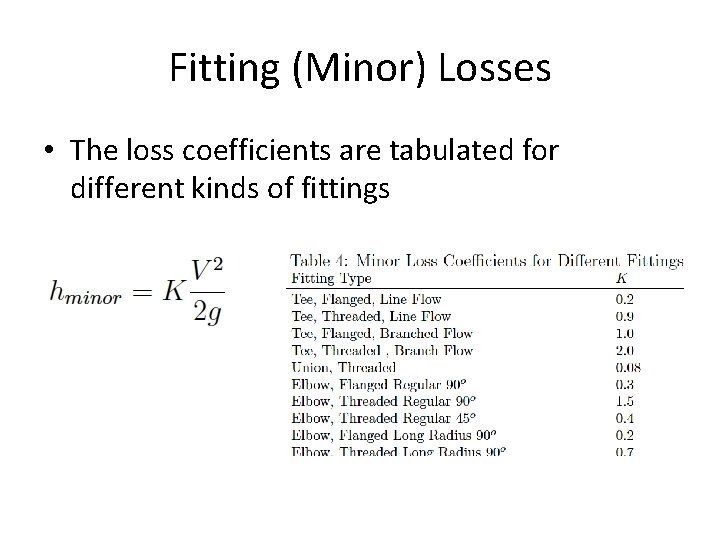Fitting (Minor) Losses • The loss coefficients are tabulated for different kinds of fittingsMoody Chart • Moody-Stanton chart is a tool to estimate the friction factor in the DW head loss model • Used for the pipe loss component of friction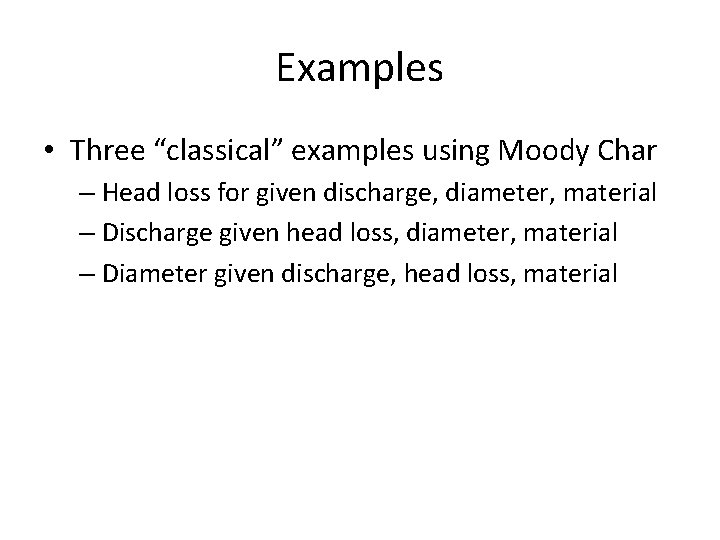Examples • Three “classical” examples using Moody Char – Head loss for given discharge, diameter, material – Discharge given head loss, diameter, material – Diameter given discharge, head loss, material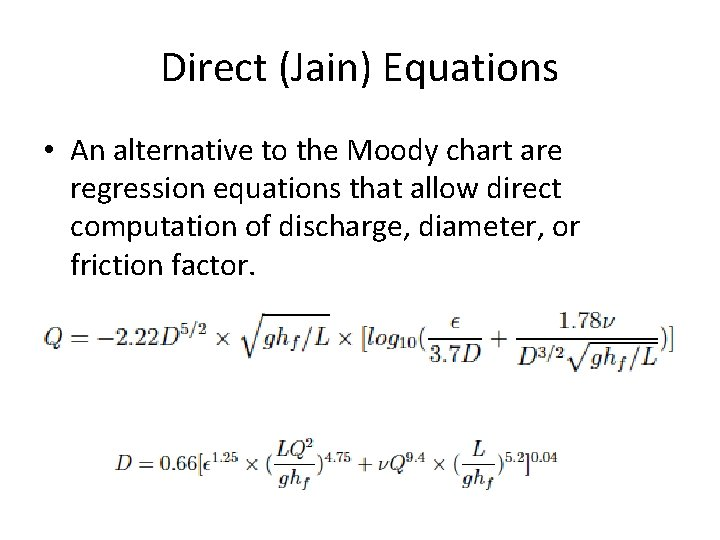Direct (Jain) Equations • An alternative to the Moody chart are regression equations that allow direct computation of discharge, diameter, or friction factor.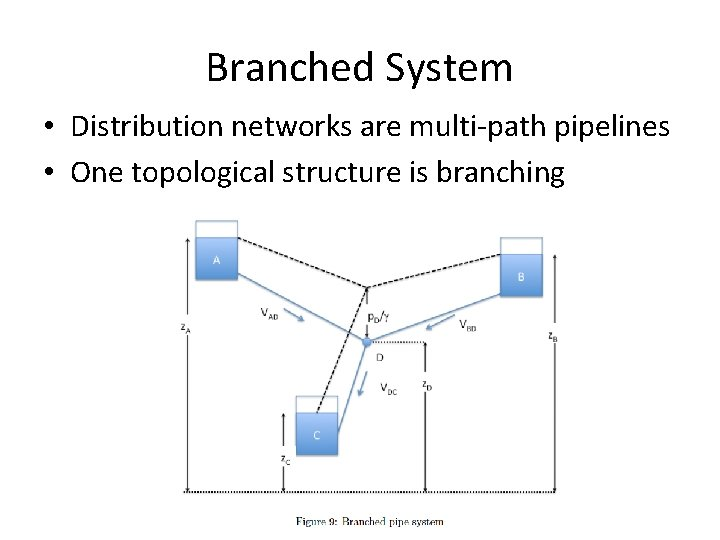Branched System • Distribution networks are multi-path pipelines • One topological structure is branching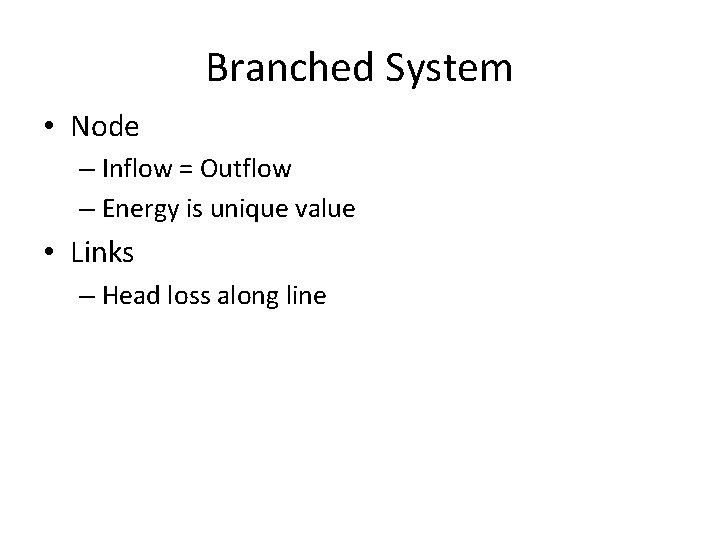Branched System • Node – Inflow = Outflow – Energy is unique value • Links – Head loss along line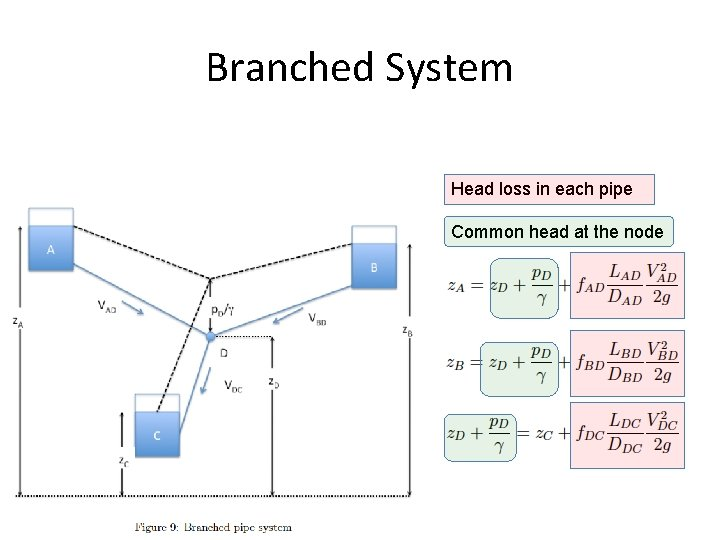Branched System Head loss in each pipe Common head at the node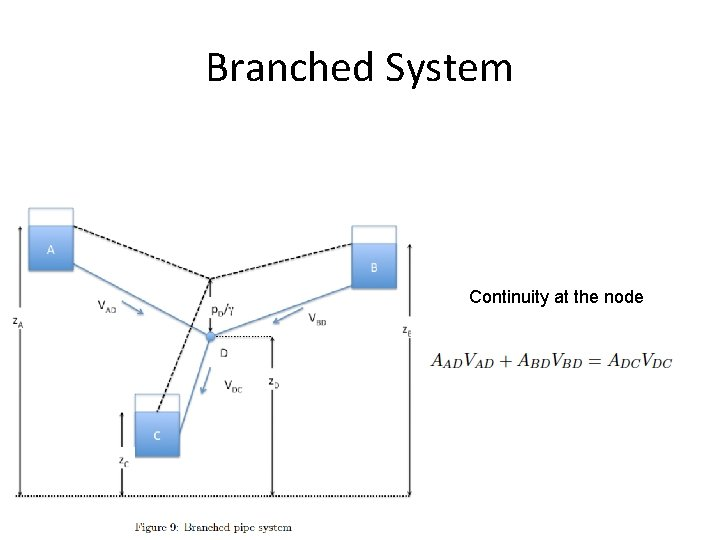Branched System Continuity at the node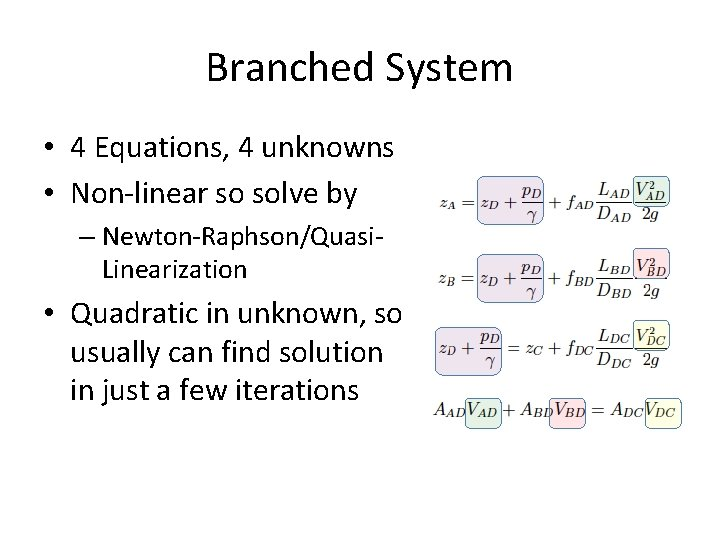Branched System • 4 Equations, 4 unknowns • Non-linear so solve by – Newton-Raphson/Quasi. Linearization • Quadratic in unknown, so usually can find solution in just a few iterationsLooped System • Looped system is extension of branching where one or more pipes rejoin at a different node.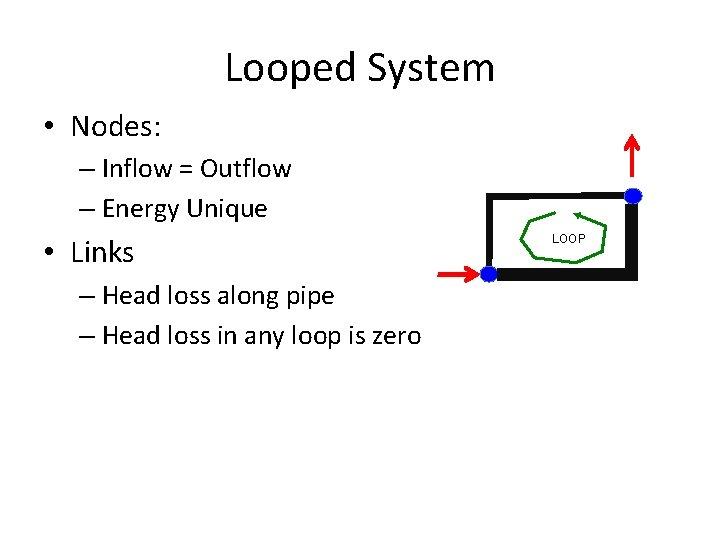Looped System • Nodes: – Inflow = Outflow – Energy Unique • Links – Head loss along pipe – Head loss in any loop is zero LOOPExamples • Branched System • Loop SystemHydraulic Grade Line • Hydraulic grade line is a plot along a conduit profile of the sum of elevation and pressure head at a location. • It is where a free surface would exist if there were a piezometer installed in the pipelineEnergy Grade Line • Hydraulic grade line is a plot along a conduit profile of the sum of elevation, pressure, and velocity head at a location.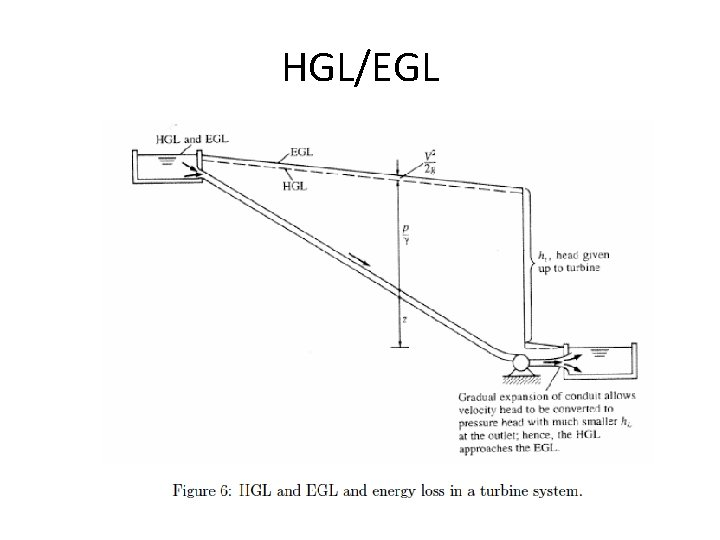HGL/EGL